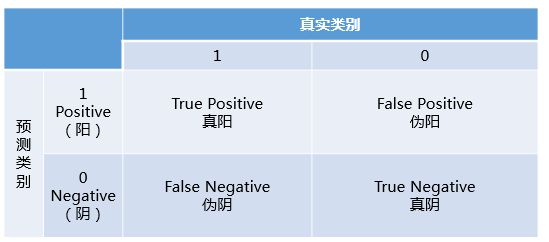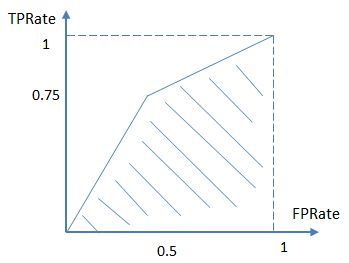# 导航

## 1.错误率和准确率

$E(f;D)=\frac{1}{m}\sum_{i=1}^m\prod(f(x_i)!=y_i)$

## 2.查准率，查全率与F1$P=\frac{TP}{TP+FP}$

$R=\frac{TP}{TP+FN}$

F1是基于P和R的调和平均值，定义为：$\frac{1}{F_1}=\frac{1}{2}(\frac{1}{P}+\frac{1}{R})$,是一种在查全率和查准率之间的权衡。

## 3 ROC和AUC

$TPRate = \frac{TP}{TP+FN}$

$FPRate = \frac{FP}{FP+TN}$

TPR与上一节的查全率其实是一个概念，TPR的意义是在所有的真实类别为1的样本中，预测类别为1的比例。FPR的意义是所有真实类别为0的样本中，预测类别为1的比例。## 4 MSE和RMSE

posted on 2018-07-04 12:26  醉月风纯  阅读(201)  评论(0编辑  收藏  举报